# Python Operators

## Python Programming Training Certification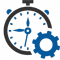Flexible Hours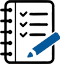100 Assignments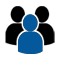Instructor Led online Training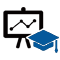50 LMS Access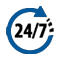24X7 Support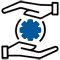100% Skill Level

### Enquire Now

4.9 out of 1000+ Ratings
Best Python Institute for Learning Python Course & Training, Live Project Training in Python with Django, Data Science and AI, Interview Assistance, Expert Coaching Trainers. Python Certification & Interview Assistance! Get free demo now!

### Course Overview

Python is one of the world’s top programming languages used today and Python training has become the most popular training across individuals. Training Basket’s Python Training & Certification course covers basic and advanced Python concepts and how to apply them in real-world applications.Python is a flexible and powerful open-source language that is easy to learn and consists of powerful libraries for data analysis and manipulation. Our Python training course content is curated by experts as per the standard Industry curriculum. The curriculum, coding challenges and real-life problems cover data operations in Python, strings, conditional statements, error handling, shell scripting, web scraping and the commonly used Python web framework Django. Take this Python training and certification course and become job-ready now.### Python Operators

The operator can be defined as a symbol which is responsible for a particular operation between two operands. Operators are the pillars of a program on which the logic is built in a specific programming language. Python provides a variety of operators, which are described as follows.

• Arithmetic operators
• Comparison operators
• Assignment Operators
• Logical Operators
• Bitwise Operators
• Membership Operators
• Identity Operators

Arithmetic Operators

Arithmetic operators are used to perform arithmetic operations between two operands. It includes + (addition), – (subtraction), *(multiplication), /(divide), %(reminder), //(floor division), and exponent (**) operators.

Consider the following table for a detailed explanation of arithmetic operators.

Operator Description
+ (Addition) It is used to add two operands. For example, if a = 20, b = 10 => a+b = 30
– (Subtraction) It is used to subtract the second operand from the first operand. If the first operand is less than the second operand, the value results negative. For example, if a = 20, b = 10 => a – b = 10
/ (divide) It returns the quotient after dividing the first operand by the second operand. For example, if a = 20, b = 10 => a/b = 2.0
* (Multiplication) It is used to multiply one operand with the other. For example, if a = 20, b = 10 => a * b = 200
% (reminder) It returns the reminder after dividing the first operand by the second operand. For example, if a = 20, b = 10 => a%b = 0
** (Exponent) It is an exponent operator represented as it calculates the first operand power to the second operand.
// (Floor division) It gives the floor value of the quotient produced by dividing the two operands.

Comparison operator

Comparison operators are used to comparing the value of the two operands and returns Boolean true or false accordingly. The comparison operators are described in the following table.

Operator Description
== If the value of two operands is equal, then the condition becomes true.
!= If the value of two operands is not equal, then the condition becomes true.
<= If the first operand is less than or equal to the second operand, then the condition becomes true.
>= If the first operand is greater than or equal to the second operand, then the condition becomes true.
> If the first operand is greater than the second operand, then the condition becomes true.
< If the first operand is less than the second operand, then the condition becomes true.

Assignment Operators

The assignment operators are used to assign the value of the right expression to the left operand. The assignment operators are described in the following table.

Operator Description
= It assigns the value of the right expression to the left operand.
+= It increases the value of the left operand by the value of the right operand
and assigns the modified value back to left operand. For example, if a = 10, b = 20 =
> a+ = b will be equal to a = a+ b and therefore, a = 30.
-= It decreases the value of the left operand by the value of the right
operand and assigns the modified value back to left operand. For example,
if a = 20, b = 10 => a- = b will be equal to a = a- b and therefore, a = 10.
*= It multiplies the value of the left operand by the value of the right operand
and assigns the modified value back to then the left operand. For example,
if a = 10, b = 20 => a* = b will be equal to a = a* b and therefore, a = 200.
%= It divides the value of the left operand by the value of the right operand
and assigns the reminder back to the left operand. For example, if a = 20, b = 10 =
> a % = b will be equal to a = a % b and therefore, a = 0.
**= a**=b will be equal to a=a**b, for example, if a = 4, b =2, a**=b will assign 4**2 = 16 to a.
//= A//=b will be equal to a = a// b, for example, if a = 4, b = 3, a//=b will assign 4//3 = 1 to a.

Bitwise Operators

The bitwise operators perform bit by bit operation on the values of the two operands. Consider the following example.

For example,

• if a = 7
b = 6
then, binary (a) = 0111
binary (b) = 0011

hence, a & b = 0011
a | b = 0111
a ^ b = 0100
~ a = 1000

Operator Description
& (binary and) If both the bits at the same place in two operands are 1, then 1 is copied to the result. Otherwise, 0 is copied.
| (binary or) The resulting bit will be 0 if both the bits are zero; otherwise, the resulting bit will be 1.
^ (binary xor) The resulting bit will be 1 if both the bits are different; otherwise, the resulting bit will be 0.
~ (negation) It calculates the negation of each bit of the operand, i.e., if the bit is 0, the resulting bit will be 1 and vice versa.
<< (left shift) The left operand value is moved left by the number of bits present in the right operand.
>> (right shift) The left operand is moved right by the number of bits present in the right operand.

Logical Operators

The logical operators are used primarily in the expression evaluation to make a decision. Python supports the following logical operators.

Operator Description
and If both the expression are true, then the condition will be true. If a and b
are the two expressions, a → true, b → true => a and b → true.
or If one of the expressions is true, then the condition will be true. If a and b
are the two expressions, a → true, b → false => a or b → true.
not If an expression a is true, then not (a) will be false and vice versa.

Membership Operators

Python membership operators are used to check the membership of value inside a Python data structure. If the value is present in the data structure, then the resulting value is true otherwise it returns false.

Operator Description
in It is evaluated to be true if the first operand is found in the second operand (list, tuple, or dictionary).
not in It is evaluated to be true if the first operand is not found in the second operand (list, tuple, or dictionary).

Identity Operators

The identity operators are used to decide whether an element certain class or type.

Operator Description
is It is evaluated to be true if the reference present at both sides point to the same object.
is not It is evaluated to be true if the reference present at both sides do not point to the same object.

Operator Precedence

The precedence of the operators is essential to find out since it enables us to know which operator should be evaluated first. The precedence table of the operators in Python is given below.

Operator Description
** The exponent operator is given priority over all the others used in the expression.
~ + – The negation, unary plus, and minus.
* / % // The multiplication, divide, modules, reminder, and floor division.
+ – Binary plus, and minus
>> << Left shift. and right shift
& Binary and.
^ | Binary xor, and or
<= < > >= Comparison operators (less than, less than equal to, greater than, greater then equal to).
<> == != Equality operators.
= %= /= //= -= +=
*= **=
Assignment operators
is is not Identity operators
in not in Membership operators
not or and Logical operators

### Recently Trained Students#### Jessica Biel

– Infosys

My instructor had sound Knowledge and used to puts a lot of effort that made the course as simple and easy as possible. I was aiming for with the help of the ZebLearn Online training imparted to me by this organization.#### Richard Harris

– ITC

I got my training from Zeblearn in the Python Certification Training, I would like to say that say he is one of the best trainers. He has not even trained me but also motivated me to explore more and the way he executed the project, in the end, was mind-blowing.

### Candidate’s Journey During Our Training Program#### Expert’s Advice & Selection of Module

Choosing the right type of module for the training is half the battle & Our Team of experts will help & guide you.#### Get Trained

Get Trained & Learn End to End Implementation from our Expert Trainer who are working on the same domain.#### Work on Projects

We Do make our student’s work on multiple case studies , scenario based tasks & projects in order to provide real-time exposure to them.#### Placements

We have a dedicated placement cell in order to provide placement assistance & relevant interviews to our candididates till selection